Analog to Digital Converter

From the name itself it is clear that it is a converter which converts the analog (continuously variable) signal to digital signal. This is really an electronic integrated circuit which directly converts the continuous form of signal to discrete form. It can be expressed as A/D or A-to-D or A-D or ADC. The input (analog) to this system can have any value in a range and are directly measured. But for output (digital) of an N-bit A/D converter, it should have only 2N discrete values. This A/D converter is a linkage between the analog (linear) world of transducers and discreet world of processing the signal and handling the data. The digital to analog converter (DAC) carry out the inverse function of the ADC. The schematic representation of ADC is shown below.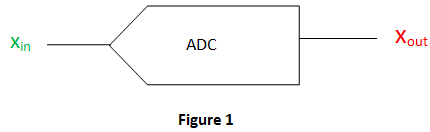There are mainly two steps involves in the process of conversion. They are

• Sampling and Holding
• Quantizing and Encoding

The whole ADC conversion process is shown in figure 2.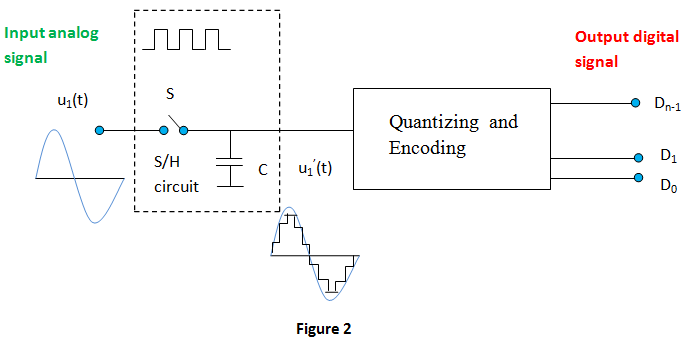Sampling and Holding

In the process of Sample and hold (S/H), the continuous signal will gets sampled and freeze (hold) the value at a steady level for a particular least period of time. It is done to remove variations in input signal which can alter the conversion process and thereby increases the accuracy. The minimum sampling rate has to be two times the maximum data frequency of the input signal.

Quantizing and Encoding

For understanding quantizing, we can first go through the term Resolution used in ADC. It is the smallest variation in analog signal that will result in a variation in the digital output. This actually represents the quantization error.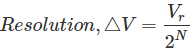V → Reference voltage range
2N → Number of states
N → Number of bits in digital output

Quantizing: It is the process in which the reference signal is partitioned into several discrete quanta and then the input signal is matched with the correct quantum.

Encoding: Here; for each quantum, a unique digital code will be assigned and after that the input signal is allocated with this digital code. The process of quantizing and encoding is demonstrated in the table below.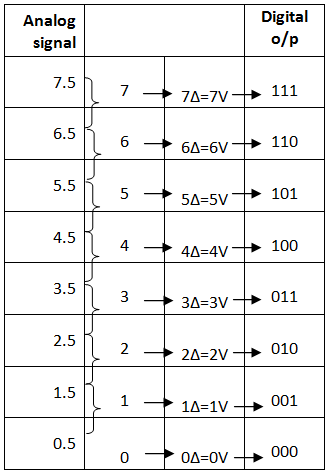From the above table we can observe that only one digital value is used to represent the whole range of voltage in an interval. Thus, an error will occur and it is called quantization error. This is the noise introduced by the process of quantization. Here the maximum quantization error is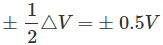Two important methods are used for improving the accuracy in ADC. They are by increasing the resolution and by increasing the sampling rate. This is shown in figure below (figure 3).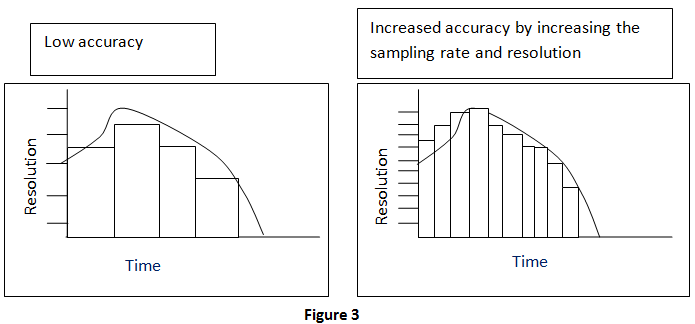Types of Analog to Digital Converter

• Successive Approximation ADC: This converter compares the input signal with the output of an internal DAC at each successive step. It is the most expensive type.
• Dual Slope ADC: It have high accuracy but very slow in operation.
• Pipeline ADC: It is same as that of two step Flash ADC.
• Delta-Sigma ADC: It has high resolution but slow due to over sampling.
• Other: Staircase ramp, Voltage-to-Frequency, Switched capacitor, tracking, Charge balancing, and resolver.

• Used together with the transducer.
• Used in computer to convert the analog signal to digital signal.
• Used in cell phones.
• Used in microcontrollers.
• Used in digital signal processing.
• Used in digital storage oscilloscopes.
• Used in scientific instruments.
• Used in music reproduction technology etc.
Want To Learn Faster? 🎓
Get electrical articles delivered to your inbox every week.
No credit card required—it’s 100% free.